Next: B Numerical Integration of Up: A Muonium Spin Polarization Previous: A.1 Transverse Field

# A.2 Longitudinal Field Polarization

In the other case of interest the initial muon polarization is along the magnetic field direction. We are then interested in the time dependence of the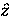component of the muon's spin in longitudinal field (LF). Since muons are produced (usually) from the decay of a charged pion (essentially at rest near the surface of the pion production target) into a two body final state via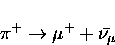(20)
and since neutrinos always have negative helicity, the muon beam produced also has negative helicity. The initial polarization of the muon, having stopped in a sample, is therefore in the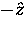direction. Again, muonium is formed with an electron captured from the medium with equal probablity of having spin up'' or down'' with respect to the quantization axis. We are interested in the matrix elements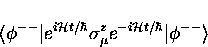(21)
and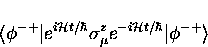(22)

The first muonium state is an eigenfunction of the Hamiltonian and the matrix element given by Eq. (A.48) is therefore simply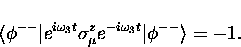(23)

Equation (A.49) can be written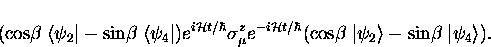(24)
Thus, using Eq. (A.17) once again,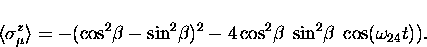(25)
Noting that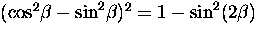and that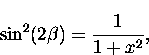thjis can be written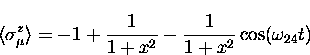(26)
where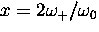.

The total muon polarization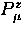in longitudinal field is then the sum of these two parts, with muonium formed with equal probability in each state, giving

The frequency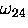in Eq. (A.54) is sufficiently high that in most experimental situations, at this time, it is not directly observable in the data as an oscillatory signal, so this term is effectively averaged to zero by the timing resolution of the spectrometer.

Thoughout this calculation we have not considered any relaxation of the muonium spin polarization.Next: B Numerical Integration of Up: A Muonium Spin Polarization Previous: A.1 Transverse Field